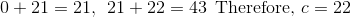# PSAT Math : How to find the answer to an arithmetic sequence

## Example Questions

### Example Question #1 : How To Find The Answer To An Arithmetic Sequence

-27, -24, -21, -18…

In the sequence above, each term after the first is 3 greater than the preceding term. Which of the following could not be a value in the sequence?

501

657

126

461

461

Explanation:

All of the values in the sequence must be a multiple of 3. All answers are multiples of 3 except 461 so 461 cannot be part of the sequence.

### Example Question #1 : Arithmetic Sequences

m, 3m, 5m, ...

The first term in the above sequence is m, and each subsequent term is equal to 2m + the previous term. If is an integer, then which of the following could NOT be the sum of the first four terms in this sequence?

80

-32

60

48

16

60

Explanation:

The fourth term of this sequence will be 5m + 2m = 7m. If we add up the first four terms, we get m + 3m + 5m + 7m = 4m + 12m = 16m. Since m is an integer, the sum of the first four terms, 16m, will have a factor of 16. Looking at the answer choices, 60 is the only answer where 16 is not a factor, so that is the correct choice.

### Example Question #3 : How To Find The Answer To An Arithmetic Sequence

The tenth term in a sequence is 40, and the twentieth term is 20. The difference between consequence terms in the sequence is constant. Find n such that the sum of the first n numbers in the sequence equals zero.

40
59
58
60
30
Explanation:

Let d represent the common difference between consecutive terms.

Let an denote the nth term in the sequence.

In order to get from the tenth term to the twentieth term in the sequence, we must add d ten times.

Thus a20 = a10 + 10d

20 = 40 + 10d

d = -2

In order to get from the first term to the tenth term, we must add d nine times.

Thus a10 = a1 + 9d

40 = a1 + 9(-2)

The first term of the sequence must be 58.

Our sequence looks like this: 58,56,54,52,50…

We are asked to find the nth term such that the sum of the first n numbers in the sequence equals 0.

58 + 56 + 54 + …. an = 0

Eventually our sequence will reach zero, after which the terms will become the negative values of previous terms in the sequence.

58 + 56 + 54 + … 6 + 4 + 2 + 0 + -2 + -4 + -6 +….-54 + -56 + -58 = 0

The sum of the term that equals -2 and the term that equals 2 will be zero. The sum of the term that equals -4 and the term that equals 4 will also be zero, and so on.

So, once we add -58 to all of the previous numbers that have been added before, all of the positive terms will cancel, and we will have a sum of zero. Thus, we need to find what number -58 is in our sequence.

It is helpful to remember that a= a+ d(n-1), because we must add d to aexactly n-1 times in order to give us an. For example, a5 = a1 + 4d, because if we add d four times to the first term, we will get the fifth term. We can use this formula to find n.

-58 = an = a1 + d(n-1)

-58 = 58 + (-2)(n-1)

n = 59

### Example Question #4 : How To Find The Answer To An Arithmetic Sequence

The first term of a sequence is 1, and every term after the first term is –2 times the preceding term. How many of the first 50 terms of this sequence are less than 5?

64

16

8

27

54

27

Explanation:

We can see how the sequence begins by writing out the first few terms:

1, –2, 4, –8, 16, –32, 64, –128.

Notice that every other term (of which there are exactly 50/2 = 25) is negative and therefore less than 25. Also notice that after the fourth term, every term is greater in absolute value than 5, so we just have to find the number of positive terms before the fourth term that are less than 5 and add that number to 25 (the number of negative terms in the first 50 terms).

Of the first four terms, there are only two that are less than 5 (i.e. 1 and 4), so we include these two numbers in our count: 25 negative numbers plus an additional 2 positive numbers are less than 5, so 27 of the first 50 terms of the sequence are less than 5.

### Example Question #5 : How To Find The Answer To An Arithmetic Sequence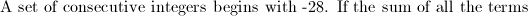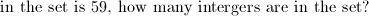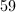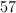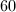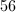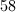Explanation:

Look for cancellations to simplify.  The sum of all consecutive integers from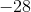tois equal to. Therefore, we must go a little farther.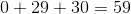, so the last number in the sequence in.  That gives usnegative integers,positive integers, and don't forget zero!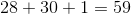.

### Example Question #1 : Arithmetic Sequences

Brad can walk 3600 feet in 10 minutes. How many yards can he walk in ten seconds?Explanation:

If Brad can walk 3600 feet in 10 minutes, then he can walk 3600/10 = 360 feet per minute, and 360/60 = 6 feet per second.

There are 3 feet in a yard, so Brad can walk 6/3 = 2 yards per second, or 2 x 10 = 20 yards in 10 seconds.

### Example Question #1 : How To Find The Answer To An Arithmetic Sequence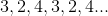If the sequence above continues as shown, what is the sum of the first 18 terms?Explanation:

The simplest way to handle this question is to consider the sequence as being a set of 3 repeating terms, (3,2,4). Within the first 18 terms in the sequence, this pattern will repeat a total of 6 times.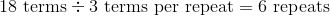The sum of one repeat is: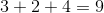Since there are 6 repeats, take the sum of one repeat (9) and multiply by the number of repeats: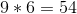The sum of the first 18 terms is 54.

### Example Question #2 : How To Find The Answer To An Arithmetic Sequence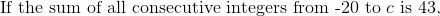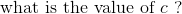Shortcut:  If you add up al the integers from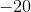to, the sum is zero because